matlab2013b

# 2.本算法理论知识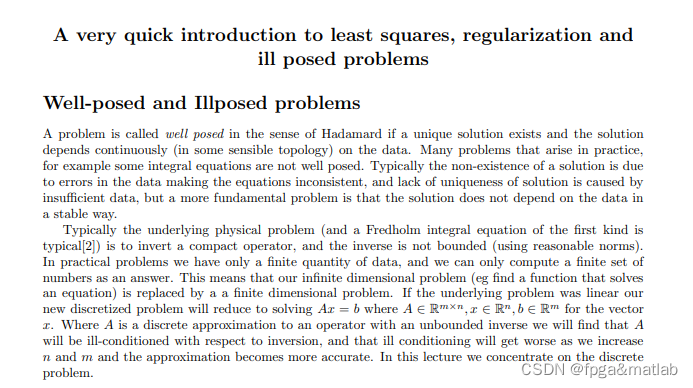# 3.部分源码

``````clc;
clear;
close all;
warning off;
pack;

%Pixel Size
Pix_Size = 32;
[I,E]    = phantom(Pix_Size);
figure;
imshow(I);

[rays,sino] = siddon(I);
ind         = find(sum(rays,2));
A           = rays(ind,:);
b           = sino(:);
b           = b(ind);

%calculate the singular value of A
s           = svds(A,size(A,2));
%plot loglog figure
figure;
subplot(121);
plot(s,'b-o');
axis([0,size(A,2),0,60]);
grid on;
axis square;
subplot(122);
loglog(s,'b-o');
axis([0,size(A,2),0,60]);
grid on;
axis square;

%Delete rows from the matrix and comment on the effect of this on the singular values
%Delete 500 rows
A2          = A;
A2(1:500,:) = [];
s2          = svds(A2,size(A2,2));

%Delete 1000 rows
A3          = A;
A3(1:1000,:)= [];
s3          = svds(A3,size(A3,2));

%Delete 2000 rows
A4           = A;
A4(1:2000,:) = [];
s4           = svds(A4,size(A4,2));

figure;
plot(s,'b');
hold on;
plot(s2,'r');
hold on;
plot(s3,'k');
hold on;
plot(s4,'g');
hold off;
axis([0,size(A,2),0,60]);
grid on;
legend('Initial singular values','singular values after delete 500 rows','singular values after delete 1000 rows','singular values after delete 2000 rows');

``````

# 4.仿真结论

课题中，这里开始说明本课题的研究工作， 我们首先需要一个SLP32*32的图片作为测试图片，这个图片可以使用MATLAB指令phantom产生。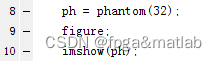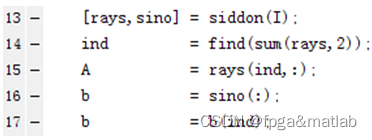通过这段代码，我们可以通过图像得到对应的稀疏矩阵A和向量b。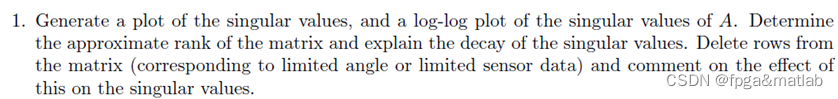main1.m

绘制奇异值曲线，用loglog坐标系来画矩阵A的奇异值图，然后计算矩阵的rank然后分析奇异值的衰减情况，将矩阵中的行删除，然后分析这个情况对奇异值的影响。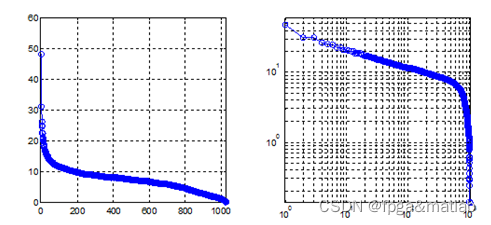然后，我们按要求，删除A中的行，然后再绘制奇异值曲线图。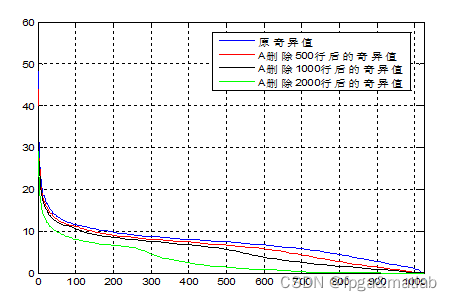从仿真结果可知，当删除rows的时候，奇异值会衰减的更快。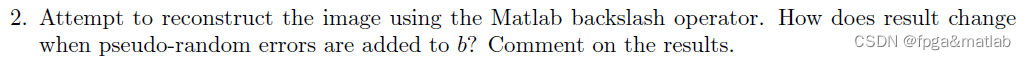main2.m

使用matlab的backslash操作来重建图像，然后分析一下结果是怎么变化的，当加入b一个随机的误差的时候。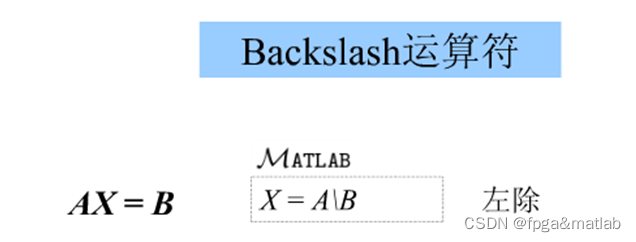首先，重建效果如下所示：

所以重建图像为I2 = A\B；PSNR = 795.3637；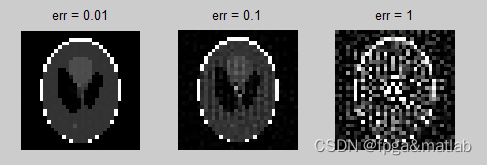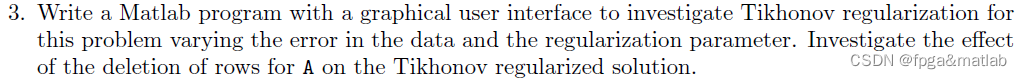main3\main3.m

运行main3.m，我们可以得到如下的界面：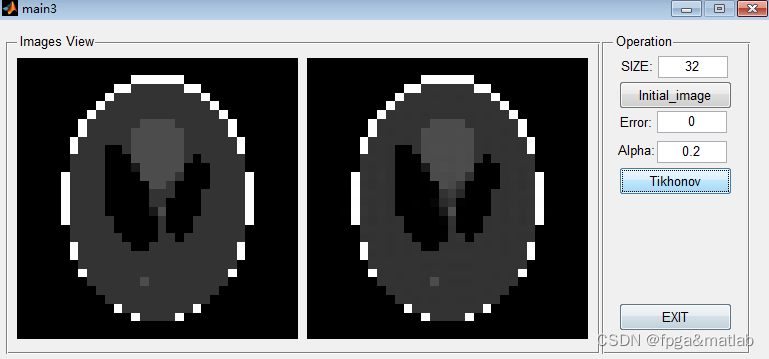# 5.参考文献

刘喜武, 刘洪, 李幼铭. 高分辨率Radon变换方法及其在地震信号处理中的应用[J]. 地球物理学进展, 2004, 19(1):8-15.A28-07